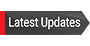Get the latest information about Fall 2021 Repopulation and COVID-19. Before coming to campus, take the COVID-19 Daily Screening.

# Faculty Research Interests

## Carmen Caprau

Dr. Caprau's research interests lie at the confluence of knot theory and abstract algebra. Knot theory now plays a large role in modern mathematics, with new directions appearing quite often. A knot is a closed curve in space that does not intersect itself, and much of knot theory is concerned with telling which knots are the same and which are different. To tell knots apart, we need knot invariants, quantities we can associate to a knot, and are unchanged no matter how we move the knot around in space. Dr. Caprau enjoy looking for new knot invariants and exploring old ones.

## Larry Cusick

Dr. Cusick's research interests include the following:
1. The group of symmetries of the hypercube.
2. Knot labelings.
3. Free actions of finite groups on topological spaces.

## Doreen De Leon

Part of Dr. De Leon's current research involves applying a wavelet transform to multigrid methods to obtain a numerical method that is more effectively and efficiently able to approximate solutions of systems of equations arising from discretizing certain steady-state diffusion equations. Diffusion equations describe how particles, heat, light, etc. spread from an area of high concentration to an area of low concentration. The steady-state diffusion problem analyzes the long-term results of diffusion.

Another area of her research involves analyzing classes of non-constant-coefficient differential equations to which certain methods for finding the particular solution may be applied. But, she is open to research in any applied mathematics area involving numerical analysis or differential equations.

## Tamas Forgacs

Dr. Forgacs' research interests involve problems in complex analysis. More specifically, he is interested in multiplier sequences, and stability preserving linear operators on the vector space R[x]. He investigates the relationship between these objects and various polynomial bases. He is also working on finding a canonical factorization for stability preserving operators.

This research area combines the use of classical analytic tools with some very recent developments in the theory of stability preserving operators. The richness of this part of mathematics can lead to research projects of varying nature, ranging from computational problems to theoretical advances.

## Oscar Vega

Dr. Vega's research is a mix of Combinatorics, Abstract Algebra, and Geometry. More precisely, his interests lay on Finite and Discrete Geometries, Graph Theory, and Algebraic Combinatorics.

## Ke Wu

Dr. Wu is a currently active researcher in the area of survival analysis. Survival analysis is a branch of statistics which deals with lifetimes in biological organisms and failure times in mechanical systems. More generally, survival analysis involves the modeling of time to event data. Typically, survival data are not fully observed due to withdrawal or termination of the study; this phenomenon is known as censoring. Dr. Wu has been conducting research on different statistical problems such as linear regression analysis, hazard rate (failure rate) estimation, two-sample scale problem, k-sample location problem, and convolution of two distributions of survival times under different models of censored survival data. He has eight research papers published refereed statistical journals in this area since 1999.